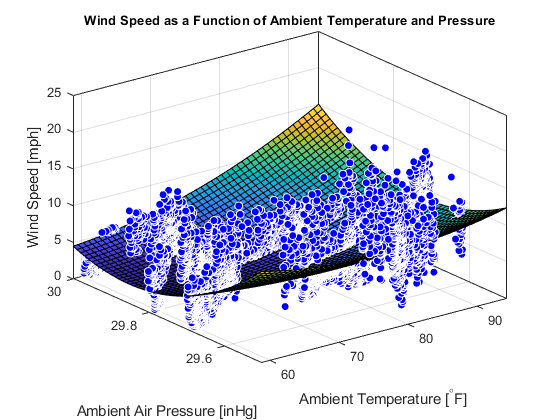# Visualize Wind Speed as a Function of Ambient Temperature and Pressure

This example shows how to visualize the variation of wind speed as a function of ambient air temperature and pressure using Curve Fitting Toolbox™. You read ThingSpeak™ data from a weather station and then use a 3D plot to visualize the data and the fit.

### Read Data from the Weather Station ThingSpeak Channel

ThingSpeak channel 12397 contains data from the MathWorks weather station, located in Natick, Massachusetts. The data is collected once a minute. Fields 2, 4, and 6 contain wind speed, temperature, and air pressure data, respectively.

```% Read the data using the |thingSpeakRead| function from channel 12397 on a particular week. For example, the week of May 1, 2018. ```
```startDate = datetime('May 1, 2018 0:0:0'); endDate = datetime('May 8, 2018 0:0:0'); data = thingSpeakRead(12397,'daterange',[startDate endDate],'Fields',[2 4 6],'outputFormat','table'); ```

### Fit a Surface to the Data

Changes in both air pressure and temperature affect wind speed. Assume that the variation in wind speed is explained by a second degree polynomial in ambient temperature and pressure. Use the `fit` function to fit a quadratic surface.

```fitObject = fit([data.TemperatureF,data.PressureHg],data.WindSpeedmph,'poly22'); ```

### Plot the Fitted Data

You can plot the fitted data to see if a quadratic surface fit captures the variation in wind speed.

```plot(fitObject,[data.TemperatureF,data.PressureHg],data.WindSpeedmph); xlabel('Ambient Temperature [^{\circ}F]'); ylabel('Ambient Air Pressure [inHg]'); zlabel('Wind Speed [mph]'); title('Wind Speed as a Function of Ambient Temperature and Pressure','FontSize',10); ```The quadratic fit appears to provide a good average for the fluctuating wind speed data. For this spring day, the wind speed is parabolic with rising pressure, but increses at larger temperatures.